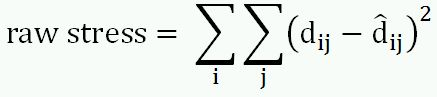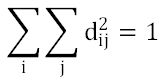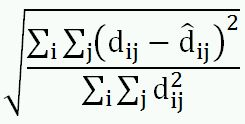## Monday, January 26, 2009

### The scaling: Basic concepts

The goal of scaling is to minimize the dissimilarity of data between the original and the reduced space. Specifically,

p_ij is the proximity (typically, dissimilarity) between object_i and object_j in the original space, whereas d_ij is the Euclidean distance between object_i and object_j in the reduced space

We use a linear regression equation to predict d_ij from p_ij, and dhat_ij is the predicted value of d_ij. Then, we want to minimize the difference between d_ij and dhat_ij, using least squares. Here, we have the raw stress index (which we want to minimize):Because the dimensions in the reduced space can be arbitrarily stretched or contracted, we normalize the raw stress index in order to achieve the following,Also, a square root places the index in the same unit as d_ij, so we have the normalized stress index (which we want to minimize):(Note. this is Kruskal's stress formula 1)

Typically, a monotone regression (aka., isotonic regression) is used instead of a linear regression, and it leads to minimizing distance ranks and therefore non-metric MDS. If a linear regression is used, it is metric MDS.

According to Kruskal and Wish (1978), with non-metric MDS, at least 9 objects are required for a 2D solution, while at least 13 objects are required for a 3D solution.

Degenerate solution:

According to Merriam-Webster dictionary, degenerate means " being mathematically simpler (as by having a factor or constant equal to zero) than the typical case".

In MDS, a degenerate solution is one with a zero (or very close to zero) stress value but retaining no (or minimal) structural information about the data. For example, the objects cluster into a few (e.g., 2) nodes and the dimensions are uninterpretable.